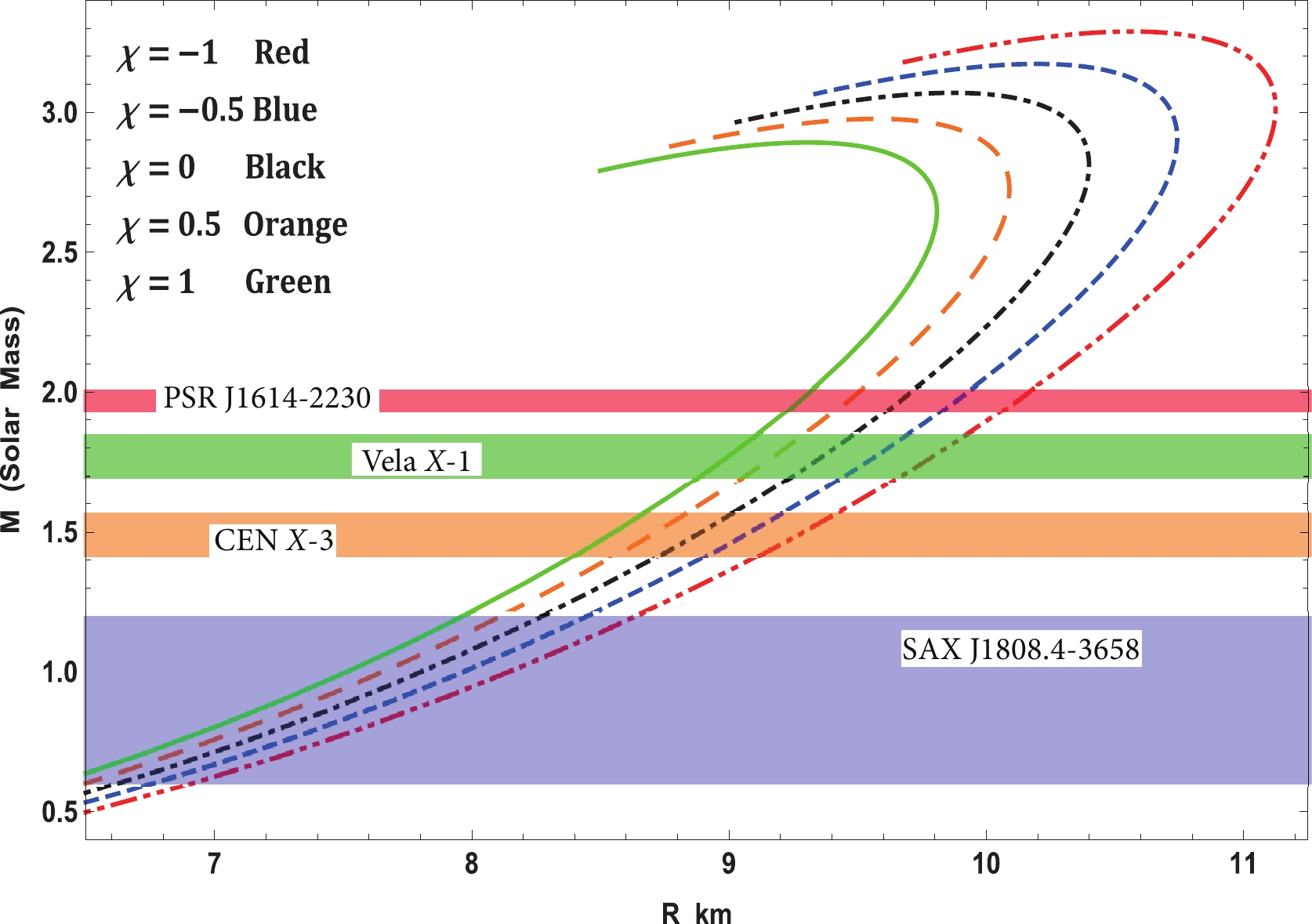# Exploring physical properties of compact stars in ${ f(R,T)}$-gravity: An embedding approach

• Solving field equations exactly in $f(R,T)-$gravity is a challenging task. To do so, many authors have adopted different methods such as assuming both the metric functions and an equation of state (EoS) and a metric function. However, such methods may not always lead to well-behaved solutions, and the solutions may even be rejected after complete calculations. Nevertheless, very recent studies on embedding class-one methods suggest that the chances of arriving at a well-behaved solution are very high, which is inspiring. In the class-one approach, one of the metric potentials is estimated and the other can be obtained using the Karmarkar condition. In this study, a new class-one solution is proposed that is well-behaved from all physical points of view. The nature of the solution is analyzed by tuning the $f(R,T)-$coupling parameter $\chi$, and it is found that the solution leads to a stiffer EoS for $\chi=-1$than that for $\chi=1$. This is because for small values of $\chi$, the velocity of sound is higher, leading to higher values of $M_{\rm max}$in the $M-R$curve and the EoS parameter $\omega$. The solution satisfies the causality condition and energy conditions and remains stable and static under radial perturbations (static stability criterion) and in equilibrium (modified TOV equation). The resulting $M-R$diagram is well-fitted with observed values from a few compact stars such as PSR J1614-2230, Vela X-1, Cen X-3, and SAX J1808.4-3658. Therefore, for different values of $\chi$, the corresponding radii and their respective moments of inertia have been predicted from the $M-I$curve.
••Get Citation
Ksh. Newton Singh, Abdelghani Errehymy, Farook Rahaman and Mohammed Daoud. Exploring physical properties of compact stars in $f(R,T)-$gravity: An embedding approach[J]. Chinese Physics C. doi: 10.1088/1674-1137/abab88
Ksh. Newton Singh, Abdelghani Errehymy, Farook Rahaman and Mohammed Daoud. Exploring physical properties of compact stars in $f(R,T)-$gravity: An embedding approach[J]. Chinese Physics C.Milestone
Article Metric

Article Views(153)
Cited by(0)
Policy on re-use
To reuse of subscription content published by CPC, the users need to request permission from CPC, unless the content was published under an Open Access license which automatically permits that type of reuse.
###### 通讯作者: 陈斌, bchen63@163.com
• 1.

沈阳化工大学材料科学与工程学院 沈阳 110142

Title:
Email:

## Exploring physical properties of compact stars in ${ f(R,T)}$-gravity: An embedding approach

###### Corresponding author: Mohammed Daoud, m_daoud@hotmail.com
• 1. Department of Physics, National Defence Academy, Khadakwasla, Pune- 411023, India
• 2. Department of Mathematics, Jadavpur University, Kolkata- 700032, India
• 3. Laboratory of High Energy Physics and Condensed Matter (LPHEMaC), Department of Physics, Faculty of Sciences Aïn Chock, University of Hassan II, B.P. 5366 Maarif, Casablanca 20100, Morocco
• 4. Department of Physics, Faculty of Sciences, University of Ibn Tofail, B.P. 133, Kenitra 14000, Morocco
• 5. Abdus Salam International Centre for Theoretical Physics, Miramare, Trieste 34151, Italy

Abstract: Solving field equations exactly in $f(R,T)-$gravity is a challenging task. To do so, many authors have adopted different methods such as assuming both the metric functions and an equation of state (EoS) and a metric function. However, such methods may not always lead to well-behaved solutions, and the solutions may even be rejected after complete calculations. Nevertheless, very recent studies on embedding class-one methods suggest that the chances of arriving at a well-behaved solution are very high, which is inspiring. In the class-one approach, one of the metric potentials is estimated and the other can be obtained using the Karmarkar condition. In this study, a new class-one solution is proposed that is well-behaved from all physical points of view. The nature of the solution is analyzed by tuning the $f(R,T)-$coupling parameter $\chi$, and it is found that the solution leads to a stiffer EoS for $\chi=-1$than that for $\chi=1$. This is because for small values of $\chi$, the velocity of sound is higher, leading to higher values of $M_{\rm max}$in the $M-R$curve and the EoS parameter $\omega$. The solution satisfies the causality condition and energy conditions and remains stable and static under radial perturbations (static stability criterion) and in equilibrium (modified TOV equation). The resulting $M-R$diagram is well-fitted with observed values from a few compact stars such as PSR J1614-2230, Vela X-1, Cen X-3, and SAX J1808.4-3658. Therefore, for different values of $\chi$, the corresponding radii and their respective moments of inertia have been predicted from the $M-I$curve.

### HTML2.   Concepts of ${ f(R, T)}-$ gravity3.   Field equations in ${ f(R,T)}-$ gravity4.   Concepts of embedding class one5.   Embedding class-one background in ${ f(R,T)}-$ gravity6.   Physical analysis of new solution7.   Boundary conditions8.   Stiffness of EoS and M-R and I-M curves9.   Discussion and conclusion
Reference (143)
PDF查看关注分享

Top

### 目录/DownLoad:  Full-Size Img  PowerPoint